HOW TO

# Convert flow accumulation lines from a DEM file to a polyline shapefile in ArcMap

Last Published: April 25, 2020

## Summary

A digital elevation model (DEM) is a raster representation of a continuous surface in which each cell represents the elevation at a location. Raster data, such as DEMs, can be converted to vector datasets to increase analytical capabilities. The line features obtained from the raster data can be used to measure the length, for example, of a road centerline or hydrology.

The instructions provided describe how to convert the flow accumulation lines from a DEM file to a polyline shapefile in ArcMap.

## Procedure

```Note:
This workflow requires the Spatial Analyst extension license.```
1. Run the Fill tool.
1. In the Catalog window, navigate to Toolboxes > System Toolboxes > Spatial Analyst Tools > Hydrology > Fill.
2. Select the DEM data as the input by clicking the drop-down arrow.
3. Specify the location of the Output flow direction raster.
4. Click OK.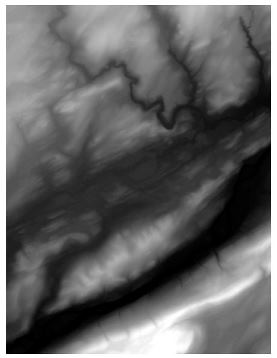```Note:
The Fill tool fills sinks in a surface raster to remove small imperfections in the DEM data.```
1. Run the Flow Direction tool.
1. Navigate to Spatial Analyst Tools > Hydrology > Flow Direction.
2. Use the output from Step 1 as the 'Input surface raster'.
3. Specify the location of the Output flow direction raster.
4. Click OK. The direction of the flow out of each cell is shown as a raster in the map.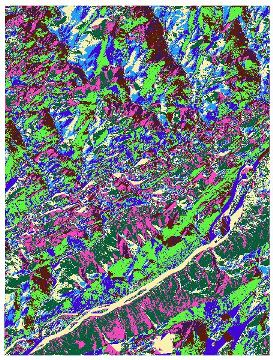```Note:
The Flow Direction tool creates a raster of flow direction from each cell to the steepest downslope neighbor.```
1. Run the Flow Accumulation tool.
1. Navigate to Spatial Analyst Tools > Hydrology > Flow Accumulation.
2. Use the output from Step 2 as the 'Input flow direction raster'.
3. Specify the location of the Output accumulation raster.
4. Click OK. The flow accumulation lines are displayed in the map.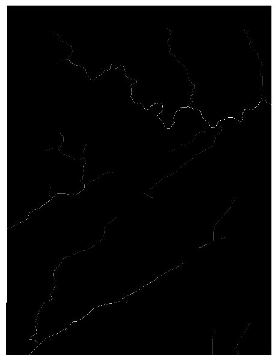1. Change the color of the accumulation lines to red.
1. Right-click the layer of the output in the Table Of Contents > Properties > Symbology tab.
2. Select Classified from the list on the left pane of the Layer Properties window.
3. Double-click the range to change the color of the flow accumulation lines to red.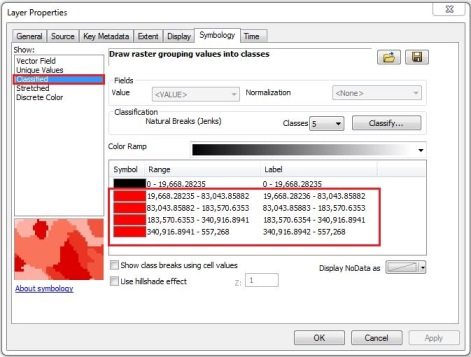```Note:
Observe the lowest value in the ranges representing the flow accumulation lines. In this example, the value is 19,668.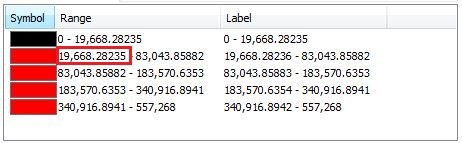```
1. Click OK. The flow accumulation lines are red.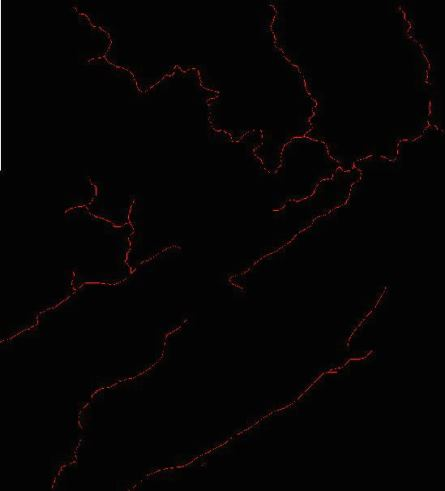1. Run the Raster Calculator tool.
1. Navigate to Spatial Analyst Tools > Map Algebra > Raster Calculator.
2. Write the following expression in the text box.
```Code:
OutRas = Raster("FlowAcc") > 19668```
```Note:
In this example, the output from Step 3 is FlowAcc and the lowest value in the ranges is 19,668.```
1. Click OK. This returns 1 for cells with a value greater than 19,668.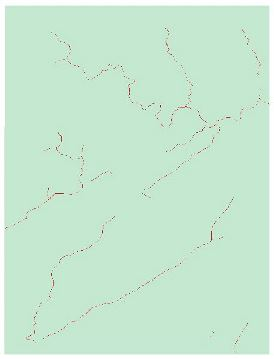1. Run the Raster To Polyline tool.
1. Navigate to Conversion Tools > From Raster > Raster to Polyline.
2. Use the output from Step 4 as the input, and save the output as a shapefile.
3. Click OK. The flow accumulation lines are converted to a polyline shapefile.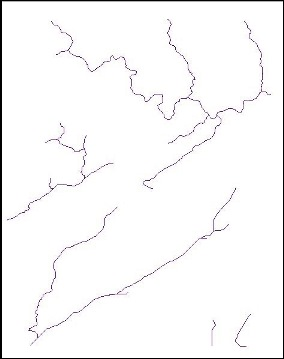Article ID:000012577

Software:
• ArcMap

Get help from ArcGIS experts

Contact technical support